Courses

# Very Short Answer Type Questions- Probability Class 10 Notes | EduRev

## Class 10 : Very Short Answer Type Questions- Probability Class 10 Notes | EduRev

The document Very Short Answer Type Questions- Probability Class 10 Notes | EduRev is a part of the Class 10 Course Class 10 Mathematics by VP Classes.
All you need of Class 10 at this link: Class 10

Q1. A letter is chosen at random from English alphabet. Find the probability that the letter chosen precedes ‘g’.

Sol. Total number of letters in English alphabet is 26.
∴ Total number of possible outcomes = 26
∵ Letters preceding ‘g’ are:
a, b, c, d, e and f
∴ Favourable outcomes = 6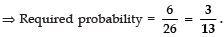Q2. A letter of English alphabet is chosen at random. Determine the probability that the letter is a consonant.

Sol. There are 26 letters in English alphabets.
⇒ Possible outcomes = 26
∵ There are 5 vowels (a, e, i, o, u) and remaining are consonants.
∴ Number of consonants = 26 – 5 = 21
⇒ Favourable outcomes = 21

∴ P(consonants)  = 21/16

Q3. A bag contains 9 black and 12 white balls. One ball is drawn at random. What is the probability that the ball drawn is black?

Sol. Total number of balls = 9 + 12 = 21
⇒ Number of possible outcomes = 21
Number of black balls = 9
⇒ Number of favourable outcomes = 9

∴ Required probability = 9/21 = 3/7.

Q4. Find the probability that a number selected from the numbers 1 to 25 which is not a prime number when each of the given number is equally likely to be selected.

Sol. Total number of given numbers = 25
Since the numbers 2, 3, 5, 7, 11, 13, 17, 19 and 23 are prime number.
There are 9 numbers.
∴ Number of numbers that are not prime = 25 − 9 = 16

∴ Number of favourable outcomes = 16
⇒ Required probability = 16/25.

Q5. A die is thrown once. Find the probability of getting an odd number.

Sol. Total number of possible outcomes = 6
[∵ Numbers 1 to 6 are marked on the faces of a die]
∵ odd numbers are 1, 3 and 5
∴ Favourable outcomes = 3

∴ Required probability = 3/6 = 1/2.

Q6. Cards each marked with one of the numbers 6, 7, 8, ....., 15 and placed in a box and mixed thoroughly. One card is drawn at random from the box. What is the probability of getting a card with number less than 10?

Sol. ∵ There are 10 cards. ∴ Total number of possible outcomes = 10
Cards marked with a number less than 10 are: 6, 7, 8 and 9
i.e. The number of favourable outcomes = 4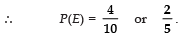Q7. A card is drawn at random from a wellshuffled deck of 52 cards. What is the probability of getting a black king?

Sol. ∵ Total number of cards = 52
∴ Number of possible outcomes = 52
Number of black king = 2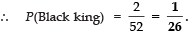Q8. What is the probability that two different friends have different birthdays? (Ignoring leap year).

Sol. Number of days in a year = 365
⇒ Number of possible outcomes = 365
Since they have different birthdays.
∴ Number of favourable outcomes = 365 − 1 = 364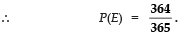Q9. A box contains 3 blue, 2 white and 4 red marbles. If a marble is drawn at random from the box, what is the probability that it will not be a white marble?

Sol. Total number of balls = 3 + 2 + 4 = 9
∴ Number of possible outcomes = 9
Since, number of white balls = 2
∴ Number of balls which are not white = 9 − 2 = 7
⇒ Number of favourable outcomes = 7

∴ P(E) = 7/9.

Q10. From a well-shuffled pack of cards, a card is drawn at random. Find the probability of getting a black queen.

Sol. ∵ Total number of cards = 52
Since, the number of black queens = 2
∴ Number of favourable outcomes = 2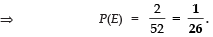Q11. A bag contains 4 red and 6 black balls. A ball is taken out of the bag at random. Find the probability of getting a black ball.

Sol. Total number of balls = 4 + 6 = 10
⇒ All possible outcomes = 10
Since, number of black balls = 6
∴ Number of favourable outcomes = 6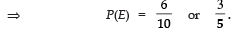Q13. A die is thrown once. Find the probability of getting a number greater than 5.

Sol. Total number of possible outcomes = 6
Since only one number i.e., 6 is greater than 5

∴ Favourable number of outcomes = 1

⇒ P(E) = 1/6.

Q14. Find the probability of obtaining 7 on a single toss of one die.

Sol. Numbers marked on a die are: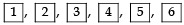∴ There are six different possible outcomes.
But none of these outcomes would produce a 7.
⇒ Favouable outcome = 0

∴ P(7) = 0/6 = 0.

When an event cannot possibly succeed, we say it is an impossible event and probability of an impossible event is zero. i.e. P(impossible event) = 0

Q15. Cards bearing numbers 3 to 20 are placed in a bag and mixed thoroughly. A card is taken out from the bag at random. What is the probability that the number on the card taken out is an even number?

Sol. Total number of cards (3 to 20) = 18
∴ Number of possible outcomes = 18
Since cards having even numbers (4, 6, 8, 10, 12, 14, 16, 18 and 20) are 9,
∴ Number of favourable outcomes = 9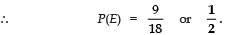Q16. Two friends were born in the year 2000. What is the probability that they have the same birthday ?

Sol. Since the year 2000 was a leap year,
∴ Total number of days in the year = 366
∵ They have the same birthday.
∴ Number of favourable outcomes = 1

⇒ P(E) = 1/36.

Q17. A box contains cards marked with numbers 5 to 20. A card is drawn from the bag at random. Find the probability of getting a number which is a perfact square.

Sol. ∵ Total number of cards = 16
∴ Possible outcomes are 16.

Since the numbers 9 and 16 are perfect numbers,
⇒ Number of favourable outcomes = 2

Offer running on EduRev: Apply code STAYHOME200 to get INR 200 off on our premium plan EduRev Infinity!

,

,

,

,

,

,

,

,

,

,

,

,

,

,

,

,

,

,

,

,

,

;